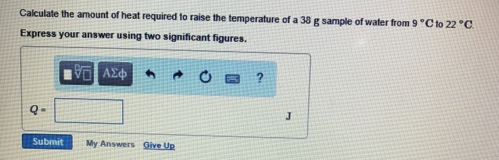Chemistry Calorimetry Solution: Calculate the amount of heat required to raise the...

# Solution: Calculate the amount of heat required to raise the temperature of a 38 g sample of water from 9°C to 22°C.

Problem

Calculate the amount of heat required to raise the temperature of a 38 g sample of water from 9°C to 22°C.View Complete Written Solution